Ex 12.2

Chapter 12 Class 10 Areas related to Circles (Term 1)
Serial order wise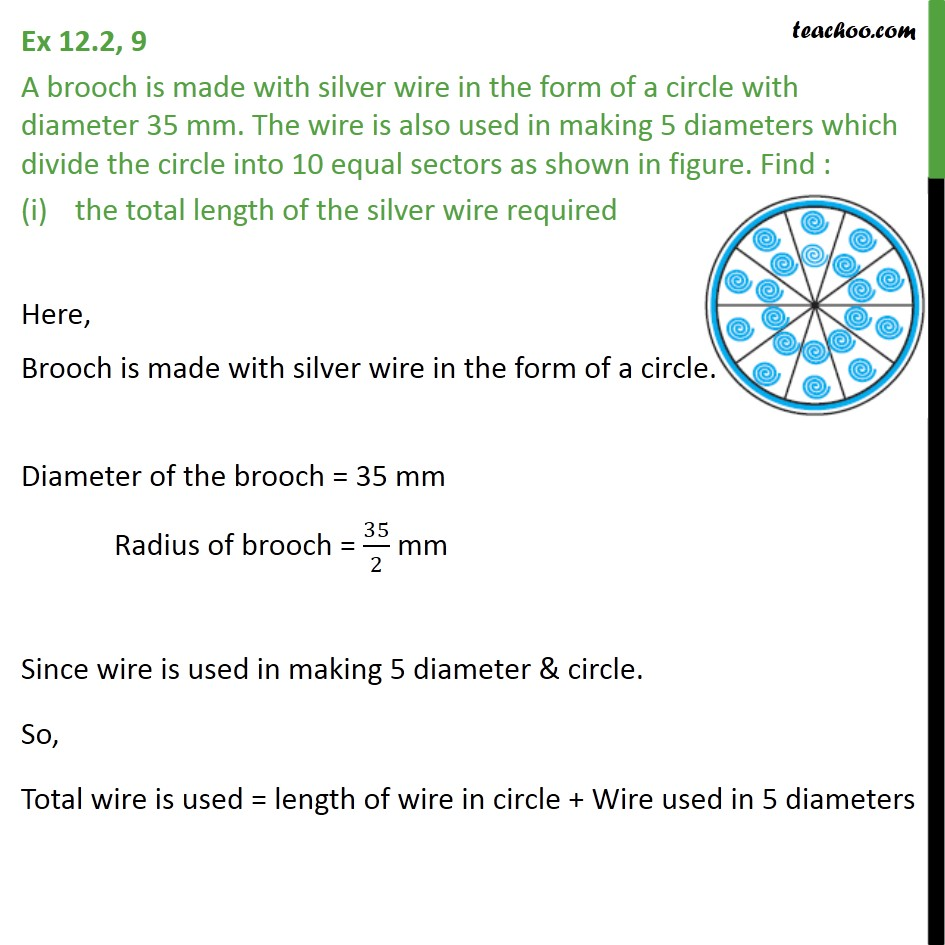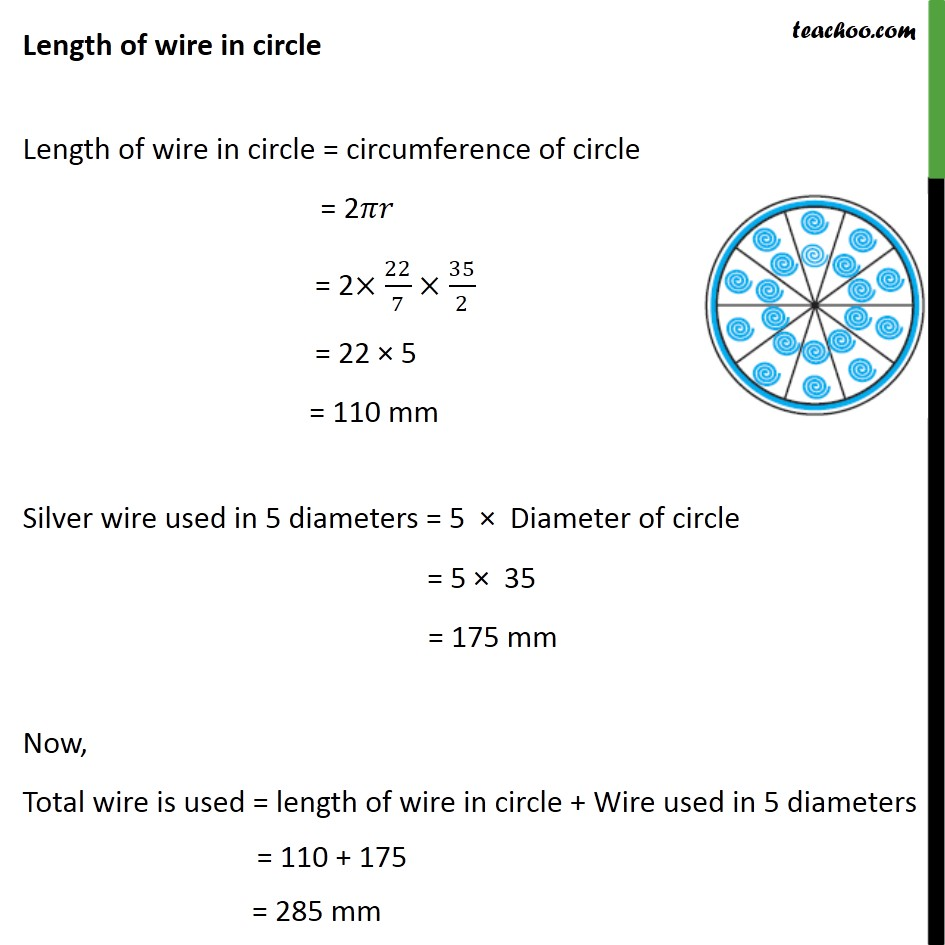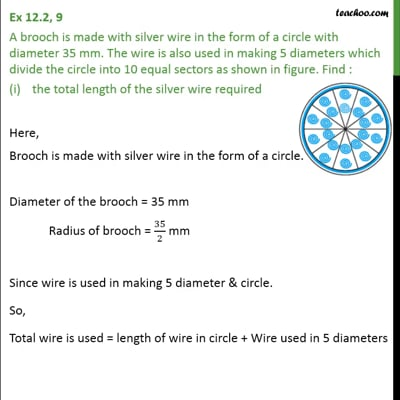This video is only available for Teachoo black users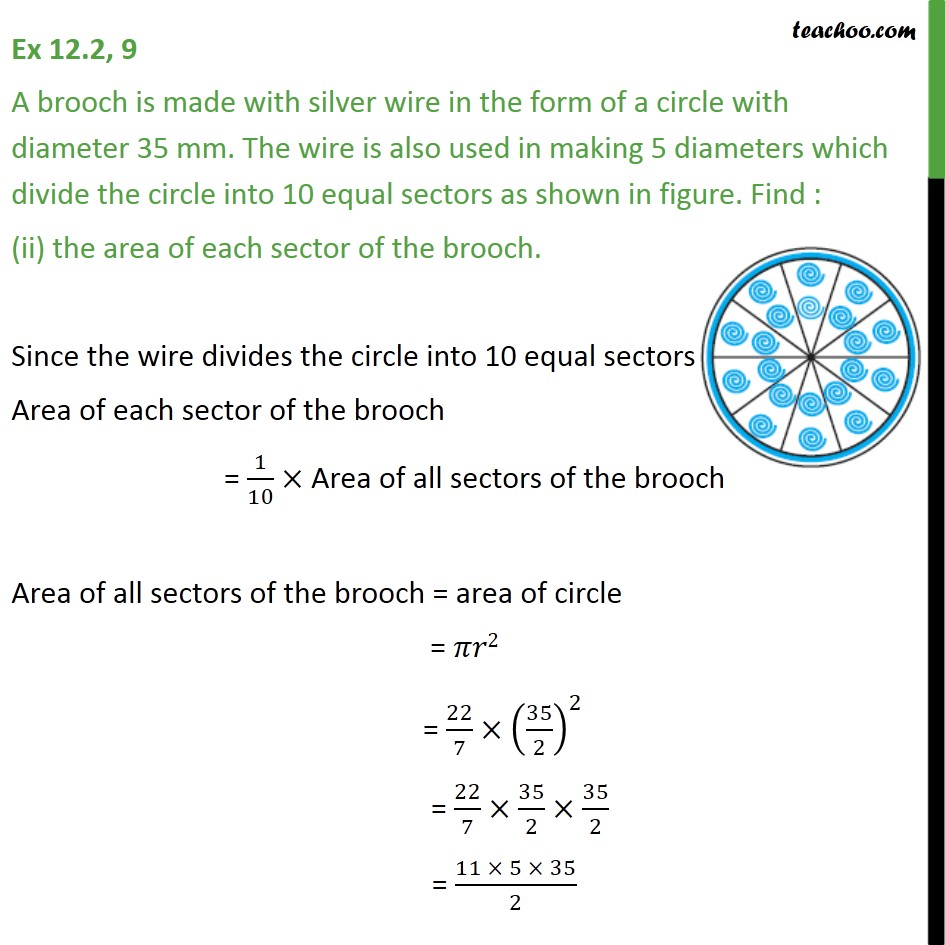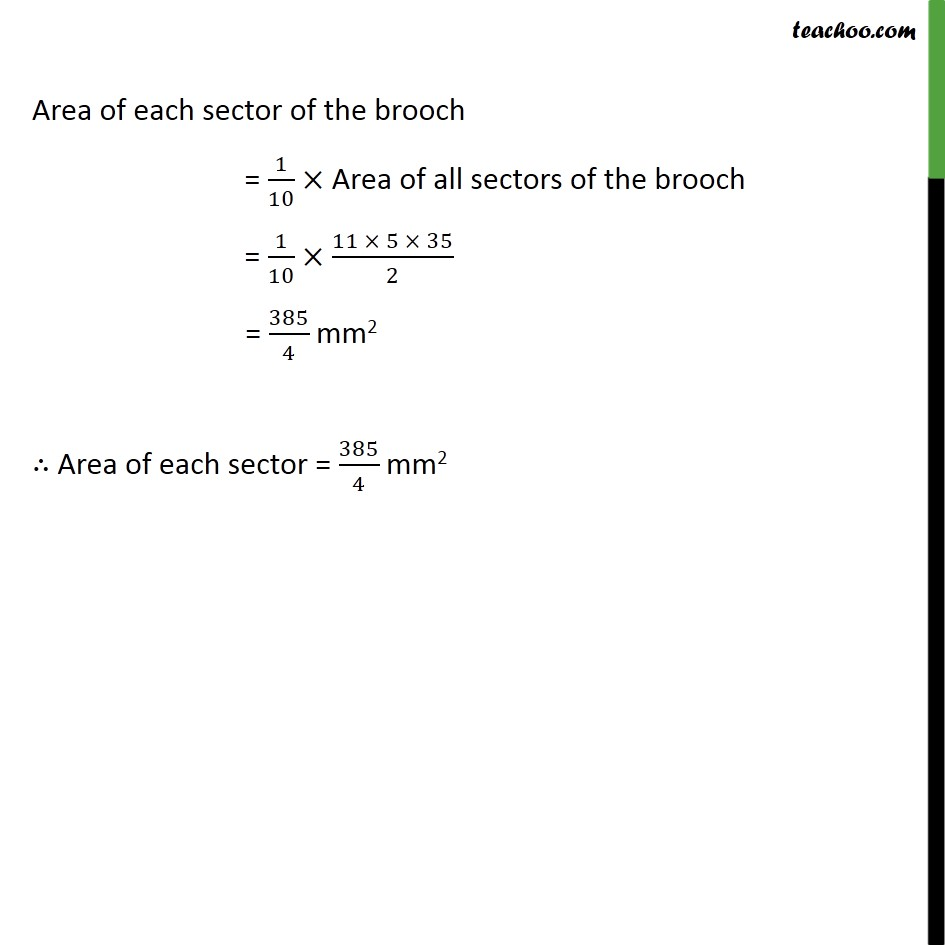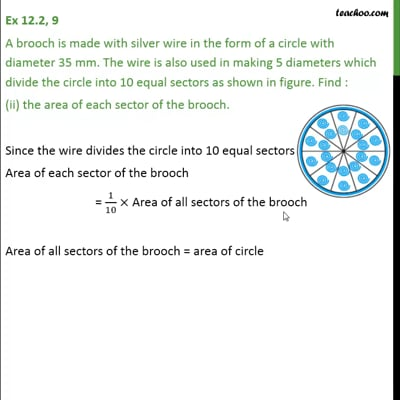This video is only available for Teachoo black users

### Transcript

Ex 12.2, 9 A brooch is made with silver wire in the form of a circle with diameter 35 mm. The wire is also used in making 5 diameters which divide the circle into 10 equal sectors as shown in figure. Find : the total length of the silver wire required Here, Brooch is made with silver wire in the form of a circle. Diameter of the brooch = 35 mm Radius of brooch = 35/2 mm Since wire is used in making 5 diameter & circle. So, Total wire is used = length of wire in circle + Wire used in 5 diameters Length of wire in circle Length of wire in circle = circumference of circle = 2 = 2 22/7 35/2 = 22 5 = 110 mm Silver wire used in 5 diameters = 5 Diameter of circle = 5 35 = 175 mm Now, Total wire is used = length of wire in circle + Wire used in 5 diameters = 110 + 175 = 285 mm Ex 12.2, 9 A brooch is made with silver wire in the form of a circle with diameter 35 mm. The wire is also used in making 5 diameters which divide the circle into 10 equal sectors as shown in figure. Find : (ii) the area of each sector of the brooch. Since the wire divides the circle into 10 equal sectors Area of each sector of the brooch = 1/10 Area of all sectors of the brooch Area of all sectors of the brooch = area of circle = 2 = 22/7 (35/2)^2 = 22/7 35/2 35/2 = (11 5 35)/2 Area of each sector of the brooch = 1/10 Area of all sectors of the brooch = 1/10 (11 5 35)/2 = 385/4 mm2 Area of each sector = 385/4 mm2